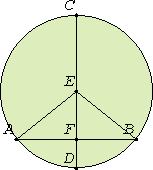# Proposition 3

If a straight line passing through the center of a circle bisects a straight line not passing through the center, then it also cuts it at right angles; and if it cuts it at right angles, then it also bisects it.

Let a straight line CD passing through the center of a circle ABC bisect a straight line AB not passing through the center at the point F.

I say that it also cuts it at right angles.III.1

Take the center E of the circle ABC, and join EA and EB.

Then, since AF equals FB, and FE is common, two sides equal two sides, and the base EA equals the base EB, therefore the angle AFE equals the angle BFE.

I.Def.10

But, when a straight line standing on another straight line makes the adjacent angles equal to one another, each of the equal angles is right, therefore each of the angles AFE and BFE is right.

Therefore CD, which passes through the center and bisects AB which does not pass through the center, also cuts it at right angles.

Next, let CD cut AB at right angles.

I say that it also bisects it, that is, that AF equals FB.

I.5

For, with the same construction, since EA equals EB, the angle EAF also equals the angle EBF.

I.26

But the right angle AFE equals the right angle BFE, therefore EAF and EBF are two triangles having two angles equal to two angles and one side equal to one side, namely EF, which is common to them, and opposite one of the equal angles. Therefore they also have the remaining sides equal to the remaining sides

Therefore AF equals FB.

Therefore if a straight line passing through the center of a circle bisects a straight line not passing through the center, then it also cuts it at right angles; and if it cuts it at right angles, then it also bisects it.

Q.E.D.

## Guide

Compare this statement to the corollary of proposition III.1.

This proposition is used in the next one, a few others in Book III, and XII.16.